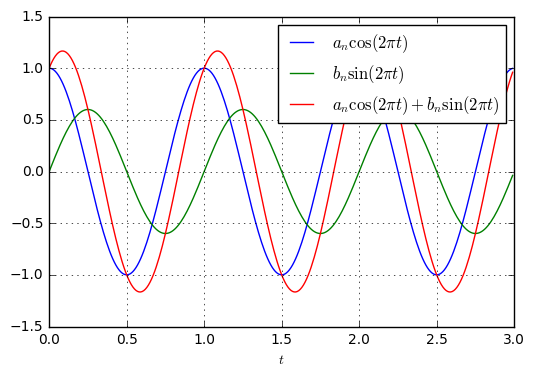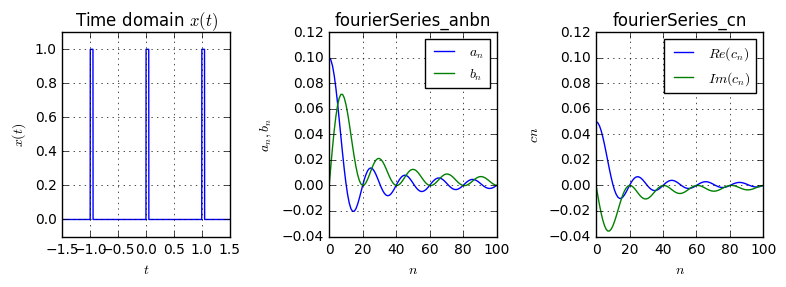# The complex Fourier Series and its relation to the Fourier Transform¶

In two recent articles we have talked about the Fourier Series and an application in harmonic analysis of instrument sounds in terms of their Fourier coefficients. In this article, we will analyze the relation between the Fourier Series and the Fourier Transform.

## The Fourier Series as sums of sines and cosines¶

To recap, the Fourier series of a signal $x(t)$ with period $P$ is given by

\begin{align}x(t)=\frac{a_0}{2}+\sum_{n=1}^\infty a_n\cos(2\pi nt/P)+b_n\sin(2\pi nt/P)\end{align}

where the coefficients are given by

\begin{align}a_n&=\frac{2}{P}\int_{-\frac{P}{2}}^\frac{P}{2}x(t)\cos(2\pi nt/P)dt\\b_n&=\frac{2}{P}\int_{-\frac{P}{2}}^\frac{P}{2}x(t)\sin(2\pi nt/P)dt\end{align}.

As we see, the Fourier series is a sum of sines and cosines with different amplitudes. Let us first look at the sum of a sine and cosine with different amplitudes:

### Sum of a sine and cosine with equal frequency¶Run code interactively  Hide
Fs = 100  # the sampling frequency for the discrete analysis
T = 3     # time duration to look at
P = 1     # signal period
t = np.arange(0, T, 1/Fs)

a_n = 1
b_n = 0.6

s = lambda t: a_n*np.cos(2*np.pi*t/P)
c = lambda t: b_n*np.sin(2*np.pi*t/P)

plt.plot(t, s(t), 'b', label='$a_n\cos(2\pi t)$')
plt.plot(t, c(t), 'g', label='$b_n\sin(2\pi t)$')
plt.plot(t, s(t)+c(t), 'r', label='$a_n\cos(2\pi t)+b_n\sin(2\pi t)$')As it appears, the sum of a sine and cosine of different amplitudes but same frequency equals another harmonic function with different amplitude and some phase shift. Hence, we can write $$a_n\cos(2\pi nt/P)+b_n\sin(2\pi nt/P) = A_n\cos(2\pi nt/P+\phi_n)$$ where $A_n$ is the amplitude and $\phi_n$ is the phase of the resulting harmonic. In the following, we will calculate the values of $A_n$ and $\phi_n$ from $a_n, b_n$. Let us start with the following identities:

\begin{align}\cos(x)&=\frac{1}{2}(\exp(jx)+\exp(-jx))\\ \sin(x)&=-\frac{j}{2}(\exp(jx)-\exp(-jx))\end{align}.

Then, we can write the sine and cosine and their sum as

\begin{align} a_n\cos(2\pi nt/P)&=\frac{a_n}{2}(\exp(j2\pi nt/P)+\exp(-j2\pi nt/P))\\ b_n\sin(2\pi nt/P)&=-\frac{jb_n}{2}(\exp(j2\pi nt/P)-\exp(-j2\pi nt/P))\\ a_n\cos(2\pi nt/P)+b_n\sin(2\pi nt/P)&=(a_n-jb_b)\frac{1}{2}\exp(j2\pi nt/P)+(a_n+jb_n)\frac{1}{2}\exp(-j2\pi nt/P) \end{align}

We can now convert the cartesian expression for $a_n-jb_n$ into the polar form by \begin{align}&&a;_n-jb_n&=A_n\exp(j\phi_n)\\ \text{with }A_n&=\sqrt{a_n^2+b_n^2} &\text{and}&&\phi_n&=\tan^{-1}(-b_n/a_n)\end{align}

Accordingly, we can reformulate the sum of sine and cosine as \begin{align}a_n\cos(2\pi nt/P)+b_n\sin(2\pi nt/P)&=A_n\frac{1}{2}(\exp(j2\pi nt/P+\phi_n)+\exp(-j(2\pi nt/P+\phi_n))\\ &=A_n\cos(2\pi nt/P+\phi_n).\end{align}

This statement eventually confirms that the sum of a sine and cosine of same frequency but different amplitude is indeed another harmonic function. Let us verify this numerically:

def sumSineCosine(an, bn):
Fs = 100
T = 3
P = 1
t = np.arange(0, T, 1/Fs)

A = np.sqrt(an**2+bn**2)
phi = np.arctan2(-bn, an)

f1 = an*np.cos(2*np.pi*t/P)
f2 = bn*np.sin(2*np.pi*t/P)

overall = A*np.cos(2*np.pi*t/P + phi)

plt.plot(t, f1, 'b', label='$x(t)=a_n\cos(2\pi nft)$')
plt.plot(t, f2, 'g', label='$y(t)=b_n\sin(2\pi nft)$')
plt.plot(t, f1+f2, 'r', label='$x(t)+y(t)$')
plt.plot(t, overall, 'ro', lw=2, markevery=Fs//(10), label='$A_n\cos(2\pi nft+\phi)$')




As we can see, the result perfectly holds.

## The Fourier Series with amplitude and phase¶

Now, let us express the Fourier Series in terms of our new formulation $$x(t)=\frac{a_0}{2}+\sum_{n=1}^\infty A_n\cos(2\pi nt/P+\phi_n)$$

Here we see, that $x(t)$ is consisting of different harmonics, with the $n$th one having the amplitude $A_n$. Since a harmonic function wave with amplitude $A$ has power $A^2/2$, the $n$th harmonic of $x(t)$ has the power $A_n^2/2=\frac{1}{2}(a_n^2+b_n^2)$.

## Fourier Series with complex exponential¶

Let us now write the Fourier Series even in a different form. By replacing the sum of sine and cosine with exponential terms, we get

\begin{align}x(t)&=\frac{a_0}{2}+\sum_{n=1}^\infty a_n\cos(2\pi nt/P)+b_n\sin(2\pi nt/P)\\ &=\frac{a_0}{2}+\sum_{n=1}^\infty \frac{a_n-jb_n}{2}\exp(j2\pi nt/P) + \frac{a_n+jb_n}{2}\exp(-j2\pi nt/P)\end{align}

Let us now set $$c_n=\begin{cases}\frac{a_n-jb_n}{2} & n > 0\\\frac{a_0}{2} & n=0 \\ \frac{a_n+jb_n}{2} & c < 0\end{cases},$$

such that we can alternatively write the Fourier series as

$$x(t)=\sum_{n=-\infty}^{\infty}c_n\exp(j2\pi nt/P).$$

Even, the calculation of the coefficients $c_n$ is very straight-forward, as we have

\begin{align}c_n = \frac{a_n-jb_n}{2}&=\frac{1}{2}\left[\frac{2}{P}\int_{-\frac{P}{2}}^{\frac{P}{2}}x(t)\cos(2\pi nt/P)dt-j\frac{2}{P}\int_{-\frac{P}{2}}^{\frac{P}{2}}x(t)\sin(2\pi nt/P)dt\right]\\&=\frac{1}{P}\int_{-\frac{P}{2}}^{\frac{P}{2}}x(t)[\cos(2\pi nt/P)-j\sin(2\pi nt/P)]dt\\&=\frac{1}{P}\int_{-\frac{P}{2}}^{\frac{P}{2}}x(t)\exp(-j2\pi nt/P)dt\end{align}

for $n>0$. We get exactly the same expression for $n\leq 0$.

So, to summarize, the formulation for the Fourier series is given by

\begin{align}x(t)&=\sum_{n=-\infty}^{\infty}c_n\exp(j2\pi nt/P)\\ \text{with }c_n&=\int_{-\frac{P}{2}}^{\frac{P}{2}}x(t)\exp(-j2\pi nt/P)dt.\end{align}

We can again verify this numerically. First, let us implement the two different possibilities to calculate the Fourier series coefficients $a_n,b_n$ or $c_n$:

def fourierSeries_anbn(period, N):
"""Calculate the Fourier series coefficients an, bn up to the Nth harmonic"""
result = []
T = len(period)
t = np.arange(T)
for n in range(N+1):
an = 2/T*(period * np.cos(2*np.pi*n*t/T)).sum()
bn = 2/T*(period * np.sin(2*np.pi*n*t/T)).sum()
result.append((an, bn))
return np.array(result)

def fourierSeries_cn(period, N):
"""Calculate the Fourier series coefficients an, bn up to the Nth harmonic"""
result = []
T = len(period)
t = np.arange(T)
for n in range(N+1):
c_plusn = 1/T * (period * np.exp(-2j*np.pi*n*t/T)).sum()
c_minusn = 1/T * (period * np.exp(2j*np.pi*n*t/T)).sum()
result.append((c_plusn, c_minusn))
return np.array(result)


Then, let's calculate the coefficients for some function $x(t)$ with both methods and compare them.

x = lambda t: (abs(t % 1)<0.05).astype(float)  # define a rectangular function

t = np.arange(-1.5, 1.5, 0.001)

plt.subplot(131)
plt.plot(t, x(t))

t_period = np.arange(0, 1, 0.001)
period = x(t_period)
anbn = fourierSeries_anbn(period, 100)
cn = fourierSeries_cn(period, 100)

plt.subplot(132)
plt.plot(anbn[:,0], label='$a_n$')
plt.plot(anbn[:,1], label='$b_n$')

plt.subplot(133)
plt.plot(cn[:,0].real, label='$Re(c_n)$')
plt.plot(cn[:,0].imag, label='$Im(c_n)$')As shown, the relation $c_n=\frac{a_n-jb_n}{2}, n>0$ exactly holds.

## The relation between the Fourier Series and Fourier Transform¶

Let us first repeat the Fourier series and Fourier transform pairs:

\begin{align}x(t)&=\sum_{n=-\infty}^{\infty}c_n\exp(j2\pi \frac{n}{P}t) &c;_n&=\int_{-\frac{P}{2}}^{\frac{P}{2}}x(t)\exp(-j2\pi \frac{n}{P}t)dt&\text{Fourier Series}\\ x(t)&=\int_{-\infty}^{\infty}X(f)\exp(j2\pi ft)dt&X;(f)&=\int_{-\infty}^{\infty}x(t)\exp(-j2\pi ft)dt&\text{Fourier Transform}\end{align}

We already see, that there is quite some similarity between the expressions for the series and transform. Let us investigate their relations:

We know that the Fourier transform can be applied for an aperiodic signal, whereas the Fourier series is used for a periodic signal with period $P$. Furthermore, we see that the Fourier transform allows the signal $x(t)$ to consist of arbitrary frequencies $f$, whereas the periodic signal $x(t)$ in the Fourier series is consisting only of harmonics of discrete frequency $f_n=\frac{n}{P}$. Let us reformulate the Fourier series with using the Dirac filter property

$$\int_{-\infty}^{\infty}x(t)\delta(t-\tau)dt=x(\tau)$$

to become

$$x(t)=\int_{-\infty}^{\infty}X(f)\exp(j2\pi \frac{n}{P}t)df \text{ with }X(f)=\sum_{n=-\infty}^{\infty}c_n\delta(f-\frac{n}{P}).$$

The expression for $x(t)$ is now equal to the inverse Fourier transform, and we can already identify $X(f)$ as the spectrum of the periodic $x(t)$. We see that $X(f)$ of the periodic signal is discrete, i.e. it is nonzero at only the harmonic frequencies $\frac{n}{P}$. The difference between the discrete frequencies is $\frac{1}{P}$, i.e. it decreases with larger period lengths. If we now eventually assume $P\rightarrow\infty$, i.e. we let the period duration of the signal become infinite, we directly end up with the expression for the Fourier transform, because

$$\lim_{P\rightarrow\infty}\sum_{n=-\infty}^{\infty}c_n\delta(f-\frac{n}{P})$$

becomes a continuous function of $f$, since the Diracs get closer and closer together, eventually merging to a smooth function (intuitively; mathematical rigorous treatment is omitted here).

Let us eventually verify this relation numerically: We take a single rectangular pulse and increase its period's length, i.e. we keep the length of the rect pulse constant, but increase the distance between the pulses, eventually leading to a single, aperiodic pulse, when the period duration becomes infinite:Run code interactively  Hide
def compareSeriesAndTransform(P):
Fs = 1000
t = np.arange(0, 100, 1/Fs)
t_period = np.arange(0, P, 1/Fs)
x_p = lambda t: (abs((t % P)-0.5) <= 0.5).astype(float)
x = lambda t: (abs(t-0.5) <= 0.5).astype(float)
plt.subplot(121)
plt.plot(t, x_p(t))

cn = fourierSeries_cn(x_p(t_period), 100)[:,0]
f_discrete = np.arange(len(cn))/P

f = np.linspace(0, Fs, len(t), endpoint=False)
X = np.fft.fft(x(t))/Fs
plt.subplot(122)
plt.plot(f, abs(X), label='Fourier Tr. of rect')
plt.stem(f_discrete, abs(cn*P), label='Fourier Series $c_n$')





As we have expected, the Fourier series provides a discrete spectrum of the periodic signal. The value of the discrete samples is equal to the value of the Fourier transform of the aperiodic signal.

## Summary¶

• The Fourier Series can be formulated in 3 ways:

\begin{align}1)\quad x(t)&=\frac{a_0}{2}+\sum_{n=1}^\infty a_n\cos(2\pi nt/P)+b_n\sin(2\pi nt/P)&a;_n&=\frac{2}{P}\int_{-\frac{P}{2}}^\frac{P}{2}x(t)\cos(2\pi nt/P)dt&b;_n&=\frac{2}{P}\int_{-\frac{P}{2}}^\frac{P}{2}x(t)\sin(2\pi nt/P)dt \\ 2)\quad x(t)&=\frac{a_0}{2}+\sum_{n=1}^\infty A_n\cos(2\pi nt/P+\phi_n)&A;_n&=\sqrt{a_n^2+b_n^2}&\phi_n&=\tan^{-1}(-b_n/a_n)\\3)\quad x(t)&=\sum_{n=-\infty}^{\infty}c_n\exp(j2\pi nt/P)&c;_n&=\int_{-\frac{P}{2}}^{\frac{P}{2}}x(t)\exp(-j2\pi nt/P)dt\end{align}

• The Fourier Transform can be understood as the limiting case of the complex Fourier series, when the period grows to infinity.Do you have questions or comments? Let's dicuss below!

### Related posts

DSPIllustrations.com is a participant in the Amazon Services LLC Associates Program, an affiliate advertising program designed to provide a means for sites to earn advertising fees by advertising and linking to amazon.com, amazon.de, amazon.co.uk, amazon.it.